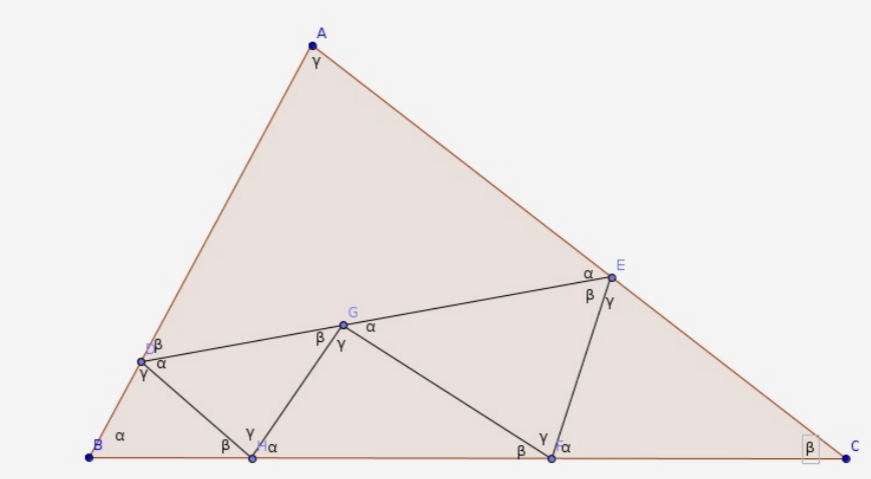# 2019字节跳动ACM程序设计冬令营题解# day1

水题/老套路题。

待补。

### 【A√】

题意：有 $$k(\leq 1000)$$ 个选手和 $$N(\leq 1000)$$ 个评委。每个评委给出一个长度为 $$k$$ 的排列，表示对每个选手的喜欢程度。选手的最终名次是这么决定的：从 $$1$$ 号评委开始，每次当前评委选取最厌恶的（且名次待定的）选手，将其设为名次待定选手中的最后一名。如果 $$n<k$$，评委循环决策，直到确定了所有选手的名次。现在你是 $$v$$ 号评委，只有你还未给出排列。你关注 $$k$$ 号选手。现在你能任意设定排列，求 $$k$$ 号选手最终排名最好能是多少。
题解：先假设没有评委 $$v$$ 模拟一遍，确定出依次被确定出的选手编号（轮到第 $$v$$ 号评委时用 $$o$$ 表示）： $$xxxxoxxxxoxxyxoxx\dots$$。设 $$y$$ 表示 $$k$$ 的位置。现在我们要找一些选手将它们填到 $$o$$ 里，使得 $$y$$ 的位置尽量靠后。首先，优先放 $$y$$ 之后的所有选手，因为这样 $$y$$ 的排名不发生变化。如果还有空格需要填 $$y$$，此时我们会发现，无论把哪个数字提前，$$y$$ 的位置必然会前进。而如果我们每次把 $$y$$ 之前的那个数提上去，$$y$$ 的位置必然前进 $$1$$ ——即这必然是最优解。所以确定 $$v$$ 号评委排列时，我们只需先把 $$x$$ 后面的数字塞进去，随后把 $$x$$ 前面的数字逆序塞进去。

### 【C√】

题意：给出一个长度小于 $$5000$$ 的字符串。将其所有子串排序并连接。有 $$M(\leq 5000)$$ 个询问，每次问新串第 $$k$$ 位的字符是什么。
题解：构出后缀自动机直接遍历的复杂度是 $$O(N^2 \sum)$$ 的，不太优美。
观察 $$height$$ 数组。假设我们在考虑 $$l \sim r$$ 这些排名的后缀。设 $$L=min(ht[l+1] \dots h[r])$$，容易发现，长度为 $$1,2,\dots,L$$ 的前缀必然依次排布在新串开头，且各有 $$r-l+1$$ 个。对于长度更长的子串，我们可以把$$(l,r)$$ 分成两半，这两半字典序严格递增。现在问题独立，先递归前一半再递归后一半。单次询问复杂度 $$O(|S|)$$，总复杂度 $$O(|S|^2+M|S|)$$

### 【D√】

题意：给出一个长度小于 $$3000$$ 的字符串，求有多少种划分方案，使得每一段字典序都是严格递增的。
题解：有个经典操作是，设 $$diff_{i,j}$$ 表示后缀 $$i$$ 和后缀 $$j$$ 的第一个不同位置，这个可以递推预处理出。倒着dp，设 $$f_{i,j}$$ 表示后缀 $$i$$ 的划分里，第一段是 $$S_{i \sim j}$$ 的方案数。转移时枚举 $$k$$，把合法的 $$f_{j+1,j}$$ 累加进答案。显然 $$k$$ 是有单调性的，可直接根据 $$diff_{i,j}$$ 计算出来，再套个前缀和优化即可。

### 【J√】

题意：给出两棵 $$2N-2(N \leq 1000)$$ 个点的树，保证每个点度数是 $$1$$$$3$$，且叶节点编号为 $$1 \sim N$$。问有多少对四元组 $$(A,B;C,D)$$，满足 $$A,B,C,D$$ 是叶子，且第一棵树上 $$A \sim B$$$$C \sim D$$ 的有交性和第二棵树上相反。
题解：转化成：求在两棵树上都不交的四元组个数。考虑枚举 $$(A,B)$$$$LCA$$ 统计个数。因为我们在无根树上考虑问题，要拓展这个 $$LCA$$ 的“定义”。严格地来说，对于一组合法的 $$(A,B,C,D)$$，定义 $$x$$$$A \sim B$$ 路径上里路径 $$C \sim D$$ 最近的点。我们每次枚举第一棵树上的 $$x$$ 和第二棵树上的 $$x$$ 并统计答案。容易发现，每个合法四元组会被统计两次。
每次枚举度数为 $$3$$ 的点。其中两个度分别放 $$A$$$$B$$，另一侧放 $$(C,D)$$（只需统计点的个数 $$t$$，方案数就是 $$C_t^2$$）。我们可以借助有根树的 $$DFS$$ 序快速维护答案。因为有两棵树，可行点数是它们的交。这是一个经典问题，对于一个点 $$x$$，假设它在两棵树上的 DFS 序是 $$(u_x,v_x)$$，将其看做一个点，每次就是矩形询问。因为是离线的，我们可以二维前缀和预处理，就支持 $$O(1)$$ 询问啦。
还有一种直接 DP 的做法：我们枚举第一棵树 $$(A,B)$$ 的 LCA。将第一个分叉的所有叶子在第二棵树的对应位置标为 $$1$$，第二个分叉标为 $$2$$，第三个分叉标为 $$3$$。对于第二棵树里的所有从 $$1$$$$2$$ 的路径，它将该树化成了若干个连通块，我们要求每个联通块 $$C_X^2$$ 的和。这可以通过比较麻烦的树形 DP $$O(N)$$ 求出来。总复杂度也是 $$O(N^2)$$

### 【K√】

题意：有一个 $$N \times N(N \leq 300)$$ 的数字矩阵。求一个子矩阵，使得 “数字和” 比上 “周长” 最大。
题解：直接做是 $$N^3 log V$$ 的：枚举上下边界后分数规划。有一个小 trick是：每次做到一组上下边界时，先去 check 一下当前答案，如果不合法直接退出二分过程。这个做法的理论复杂度是 $$O(N^3+N^2 \log V)$$ 的。
上述证明可以抽象成这么一个问题。我们有 $$N^2$$ 个箱子，每个箱子可以 $$O(N \log V)$$ 确定它的解，或者 $$O(N)$$ 验证一组解可不可行。现在要求所有箱子解的最大值。考虑一种做法：每次随机选择一个箱子确定它的最优解 $$X$$。然后遍历所有箱子 $$O(N)$$ check，如果 $$X$$ 不是该箱子的解就扔掉这个箱子。对于剩下的箱子继续这么做。这样的复杂度是 $$T(M)=N \log V+MN+T(x)$$，其中 $$x$$$$0 \sim M$$ 里随机的。事实上，这个复杂度等于 $$T(M)=N \log V+MN+T(\frac{N}{2})$$

# day2

不补了。

### 【B√】

题意：有 $$N(\leq 100000)$$ 个物品，每个物品有两维 $$(p_i,q_i)$$。现在最多可以用两次 $$split$$ 操作。每次选择一个物品和一个常数 $$p(0 < p < 1)$$，将物品按 $$p$$ 等比例拆成两个。操作完后，要将物品分成两堆，每堆两维的和都彼此相同。
题解：先考虑一维怎么做。将物品看成线段，整齐地铺在数轴上。我们在 $$\frac{sumL}{2}$$ 处切一刀，切到的那个物品需要被 $$split$$ 一次。
两维需要一点小技巧。将每个物品看做一个底边为 $$p_i$$，高为 $$\frac{q_i}{p_i}$$ 的长方形。我们需要在 $$X$$$$X+\frac{\sum p_i}{2}$$ 处切，使得中间那一块长方形的面积和是总面积的一半。如果我们按照 $$\frac{q_i}{p_i}$$ 将长方形排序，那么切出来的面积和切的位置 $$X$$ 是正相关的。二分即可，复杂度 $$O(N \log V)$$；也可以单调地扫描过去，每次解一个二次方程，复杂度 $$O(N \log N)$$

### 【C√】

题意：考虑正 $$N(\leq 5 \times 10^5)$$ 边形上的 $$N$$ 个点。将它们构成一个边权只有 $$0/1$$ 的完全图。读入 $$M$$ 条边，剩下的所有边的颜色是根据一个参入读入的函数构造的。现在要构造一个生成树，要求每条边颜色必须相同，且这些边在正 $$N$$ 边形内不能相交。
题解：最后一个限制很难，反而在暗示，存在一种很简单的构造方法。我们先考虑 $$N$$ 条环边，如果颜色全一样，显然我们直接得到了一组解（去掉任意一条边即可）。否则，必然存在一个点 $$x$$，它左右的边颜色不同。假设它相邻的两个点是 $$(u,v)$$，我们先删掉 $$x$$，连上 $$(u,v)$$，递归地构造出 $$N-1$$ 个点的生成树。最后根据生成树颜色的黑白性，再连上和 $$x$$ 相连的边即可。递归到剩下 $$2$$ 个点时必然合法。用 $$map$$ 简单维护，总复杂度 $$O(N \log N)$$

### 【D√】

题意：给出一个 $$N$$ 个点的简单多边形。对于一个定点 $$(x0,y0$$，随机枚举一条穿过它的直线。求与多边形交的长度和的期望。
题解：有一个令人惊讶的性质是：就像有向线段计算面积一样，计算长度和也是可以每条有向边独立的。对于每条有向边，积分式是形如 $$\int \frac{1}{cos(\theta)}$$ 或是 $$\int \frac{1}{sin(\theta)}$$。上下同乘一个分母即可化为多项式积分。总复杂度 $$O(N)$$

### 【E√】

题意：给出一个字符串集合 $$S_1,\dots,S_n$$。要求合法的字符串集合 $$T_j$$ 的个数，使得每一个 $$S_i$$ 能且仅能找到一个 $$j$$ 使得 $$T_j$$$$S_i$$ 的前缀。同时规定了集合大小是 $$M$$$$(|S_i| \leq 200,N,M \leq 2000)$$
题解：建出 $$S_i$$$$trie$$ 树。相当于是要在树上选择 $$M$$ 个互不相同的点，使得每个叶子仅属于一个点的子树。设 $$dp_{i,j}$$ 表示决策完了 DFS序 第 $$i$$ 个叶子，且总共选了 $$j$$ 个点的方案数。如何选当前点？暴力枚举 $$j$$ 的祖先 $$x$$，如果$$R_x=j$$，那么可以从 $$f_{L_x-1,j-1}$$ 转移过来。注意到，暴力跳父亲直到不合法的总复杂度是 $$O(VM)$$ 的（$$V$$ 是总点数），因为一个点 $$x$$ 只会被 $$R_x=j$$ 的点跳到。因为 $$V$$ 可以很大，可以建出 $$N$$ 个点的虚树，将一条链上的点压成一个。

### 【G√】

题意：在一棵 $$N(\leq 10000)$$ 个点的树上选择最多 $$K(\leq 100)$$ 条点不相交的路径，求路径上点数之和的最大值，并输出一种端点的方案。
题解：设 $$f_{x,y,0/1}$$ 表示 $$x$$ 子树里选了 $$y$$ 条链，且是否有一条链向上顶 的点数和的最大值。每次在子树里 $$dp$$ 时，是可能把两条路径并在一起的，所以转移时要设 $$G_{i,y,0/1/2}$$ 表示做到第 $$i$$ 个儿子，选了 $$y$$ 条链，且总共有 $$0/1/2$$ 三条支链向上顶的点数和的最大值。
在子树一个个dp过去的时候，再开一个数组记录一下对应方案即可还原。把 $$y$$ 限制成子树 $$size$$，复杂度就是 $$O(NK)$$ 了。题解的证明方法比较帅，分别考虑 $$size$$ 大于 $$K$$ 的和不大于 $$K$$ 的两部分，复杂度都是 $$O(NK)$$

### 【J√】

题意：定义 $$F(N)$$ 为：小于 $$N$$ 且与 $$N$$ 互质的数的和。设 $$M=F(N)$$，给出 $$M$$，求一个合法的 $$N$$$$1000$$ 组数据，$$M \leq 10^18$$
题解：$$F(N)=\prod p_i^{2k_i-1} \times (p_i-1)$$。如果我们能快速分解出 $$M$$，直接枚举选择了哪些 $$p_i$$，然后 $$O(\log M)$$ 确认即可。
直接跑 rho 太慢了。考虑先预处理出前 $$10^6$$ 的质数。对 $$M$$ 筛后，最多只会出现 $$M'=P$$$$M'=p^2$$$$M'=PQ$$ 三种形式。前两种都可以特判，终点考虑第三种。推理一下可知，$$10^6<P<Q<10^9$$，且$$Q$$ 这个质数必然出现在 $$p_i$$ 里，所以 $$P$$ 必然不出现。因为 $$P|(Q-1)$$，我们可以直接枚举 $$\frac{Q-1}{P}$$ 的值 check 即可，每次只要枚举到 $$1000$$

### 【K√】

题意：给出 $$N(\leq 250)$$ 个点，保证没有三点贡献。要在里面选出 $$5$$ 个点，$$3$$ 个构成三角形三个顶点，$$2$$ 个构成线段。要求线段和三角形严格不交，问有几种选法。

# day3

简单题。

待补。

不补了。

### 【B√】

题意：给出一个 $$N(\leq 20)$$ 个点的确定性自动机，边上是小写字母。从 $$1$$ 号点出发，每次随机找一条出边走过去，并把沿途的字母连接成字符串 $$S$$。再给出两个字符串 $$(A,B) (|A| \leq 10,|B| \leq 50)$$，任意时刻当 $$A$$$$S$$ 子串或 $$B$$$$S$$ 子序列时停止游走。问路径的期望长度，无穷大输出 $$-1$$
题解：很清晰的高斯消元题。子序列的匹配具有单调性，所以可以按 $$B$$ 串分层进行高斯消元。每层共有 $$N(|A|+1)$$ 个点，依据 $$fail$$ 数组建立方程。注意最好先判一下无穷大的情况，包括：①存在一条路径连向下一层或本层的无穷大。②本层一个封闭的圈，不连向任何已经确定的答案。

### 【C√】

题意：给出平面上的 $$N(\leq 2000)$$ 个点，求一个面积最小的三角形。
题解：先枚举三角形的最低点，将它上方的点极角排序。注意，极角序相邻的点不一定就是答案：枚举了其中一个向量后，另一个点必须是距离离它最小的。对于极角序相邻的两个 $$(x,y)$$，做一条从原点开始与其连线平行的向量 $$\gamma$$。观察到，$$x$$$$\gamma$$ 一侧的距离都优于 $$y$$，而 $$y$$ 在另一侧比较优。所以我们可以按极角序压入一些点，维护一个凸壳表示可能成为答案的点集。随着枚举的向量变化，可能会弹出凸壳顶。

### 【D√】

题意：给出一张 $$N(\leq 1000)$$ 个点，$$M(\leq 100000)$$ 条边的无向图，保证每一个点度数都一样且是偶数。现在要删掉若干边，使得剩下的图里每个点的度数都是 $$2$$
题解：考虑网络流模型：左右两排都是 $$N$$ 个点，与起点终点的限制流量都是 $$1$$；原图的一条边 $$(u,v)$$ 在正反连两次。如果满流，每个点都有一个唯一的出点，我们可以根据这个连出若干个环，问题也就解决了。
实际上还有一个小问题：这样可能会连成二元环，而二元环是不被允许的。所以我们希望原图的每条边只在网络流里被连一次，即给边定向，使得有解性不变。有一种很优美的定向方法：在原图中做一遍欧拉回路，每条边被经过的方向即为它的方向。
现在我们来证明，这种做法必然能找到解。欧拉回路完后，每个点正好有 $$k$$ 条出边和 $$k$$ 条入边。在网络流的二分图里，考虑左侧的一个集合 $$X$$，它们的出度和是 $$k|X|$$。因为右侧每个点入度也是 $$k$$，所以与 $$X$$ 关联的右侧点至少有 $$k$$ 个。由霍尔定理，这个二分图存在完备匹配。

### 【G√】

题意：将俄罗斯方块（包含所有旋转型）填入 $$4 \times N$$ 的格子中。求能填满格子的方案数。$$N \leq 10^9$$
题解：注意俄罗斯方块及其旋转型 其实就是所有四连通的网格。
一列一列填过去，最多只和前三列有关。可以状压前 $$4 \times 3$$ 的格子信息进行 dp。
有一种策略是直接暴力 $$dp$$ 1000 项，得到的数列必然是线性递推数列，找寻一下规律即可。
也可以对上限是 $$2^{12}$$ 种状态进行压缩（因为很多状态可能永远也填不满了）。比赛时直接加了些无脑剪枝，比如 "任意一个白点到右侧那一列的最短路不能超过 $$4$$"，“假设第三列的白色方块数量是 $$k$$，则左三列白色方块不能超过 $$3k$$”，"如果存在一个 $$1 \times 4$$ 的白条，先假设填上，若之后的状态不行则它也不行"。这样状态只有 $$360$$ 种左右了。再应用对称性可以缩到 $$180$$ 种，预处理转移直接矩乘。

### 【K√】

题意：$$2N$$ 个点排成一个环。要将每两人匹配成一个组，使得在环上有边的组正好有 $$K$$ 组。$$1 \times K \times N \leq 10000$$
题解：

# day4

简单题 / 码农题。

### 【B】

题意：求无穷级数 $$\sum \frac{\ln n}{n^s}$$ 的值。和标准值的绝对误差不能超过 $$1e-6$$$$1.0001 \leq s \leq 20$$ , $$s$$ 四位小数。
题解：待补。

### 【C√】

题意：给出一个字符串 $$S(|S| \leq 10^5)$$。有 $$Q(\leq 3 \times 10^5)$$ 个询问，每次给出 $$(l,r)$$，问 $$l \sim r$$ 之间有多少本质不同的子串。
题解：如果用 $$manacher$$ 求出每个位置的回文半径 $$p_i$$，那么对于一个询问 $$(l,r)$$，答案就是 $$\sum_{i=l}^r \min(p_i,i-l+1,r-i+1)$$。比赛时我想的是，每次枚举一个位置 $$i$$，去维护它对所有询问 $$(l,r)$$ 的贡献。$$min$$ 里面有 $$3$$ 个量不太好直接维护。假设我们维护好了$$(l,r)$$ 的答案，如果边界变动一格，我们可以用主席树在 $$O(\log N)$$ 的时间内求出增量（比如 $$l$$ 减一，就是询问 $$\sum_{i=l}^{(l+r)/2} [p_i<l]$$。那么我们可以分块，先用差分数组在 $$O(N \sqrt N)$$ 时间内维护出 $$f_{b,r}$$ ，表示第 $$l$$ 个块的开头到位置 $$r$$ 的答案。每次询问时移动 $$l$$ 求增量，询问复杂度 $$O(Q \sqrt N\log N)$$。但这样是过不了的。发现每次移动的询问是彼此独立的，可以先离线下来（为了防止空间不够，可以每隔几百万离线求一次），用桶排+树状数组快速求出答案，几乎可以把原来的主席树的 $$O(\log N)$$ 的常数给拿掉。
比赛中智商降了好多……反过来考虑，固定一个 $$(l,r)$$，求所有 $$p$$ 对它的贡献。 根据 $$mid$$ 划成两半，三维 $$min$$ 就直接变成了二维 $$min$$……然后离线+线段树或者直接主席树就在 $$(N \log N)$$ 时间内轻松解决了……

### 【D】

题意：给出平面直角坐标系里的三个整点表示一个三角形。现在要将其划成若干个小三角形，使得每个小三角形都和原图形相似，且大小两两不同。
题解：只有正三角形是无解（但是三个整点表示不出正三角形）。在大多数情况都可以用以下Case解决：### 【F】

题意：有一个 $$N \times M (N,M \leq 1000)$$ 的网格，$$(x,y)$$ 是障碍。用大小为 $$3$$$$L$$ 型骨牌去覆盖剩下的格子，打印一种方案或输出无解。
题解：待补。

### 【G√】

题意：图上有 $$N$$ 个点，起初没有边。有 $$M$$ 个操作，每次加入一条边 $$(u,v)$$ 或者删除一条边。每次操作完后询问桥的个数。
题解：经典的动态桥边计数问题， claris的题解 。设 $$solve(l,r,n,m)$$ 为考虑时间在 $$[l,r]$$ 的修改操作，作用范围是 $$n$$ 个点，$$m$$ 条边的图。设 $$E$$ 表示 $$[l,r]$$ 涉及到的边集，$$V$$ 表示它们两端涉及到的点集。先将 $$M-E$$ 跑一遍 $$tarjan$$，将边双缩点（边双内部的边永远不会是桥）。在剩下的树（森林）中，做出 $$V$$ 的虚树。不在虚树上的边永远都是桥，直接加入答案即可。将剩下的虚树做一张新图，递归左右两个子问题（有个细节是，虚树上的一条边要额外记录对应了原图的几条边，一旦它成为答案，这些边都是桥）。容易发现，每一层做完后，新图的点数和边数都是 $$O(r-l)$$。不计并查集复杂度，总复杂度为 $$M \log M$$
还有一种 $$M \log M \log N$$ 的 LCT 做法。将边插入时间线段树，现在只剩下加边了。用 LCT 维护当前森林，如果进来一条边 $$(u,v)$$ 所在的连通块已经连通，就在 $$u \sim v$$ 的路径上区间加 $$1$$。显然，桥的个数即为权值为 $$0$$ 的边的条数，这个可通过在 LCT 的 Splay 上维护子树最小值以及子树最小值个数来实现。注意，每次加入一条边后，只需考虑答案的增量，所以只有链上询问最小值个数，不需要带虚儿子和的 LCT。时间线段树里的回退也能很方便地实现。

### 【H√】

题意：有一棵 $$N(\leq 10^5)$$ 个点的树，每条边有边权 $$(b_i,c_i)(\leq 10^9)$$。对于一条边 $$i$$，定义 $$d_i$$ 为：考虑根到它的其它所有 $$b_j<b_i$$ 的边 $$j$$。如果不存在这样的 $$j$$，则 $$d_i=c_i$$；否则 $$d_i=median(d_j)+1$$$$median$$ 表示符合要求的边的 $$d_j$$ 的中位数。
题解：在树上DFS。套一个树状数组来满足 $$b_i$$ 这一维的限制，每个 bit 对应一棵动态开点的权值线段树（用来维护 $$d_i$$）。查询时，在符合要求的 $$log$$ 个权值线段树里一起走。时间和空间复杂度都是 $$O(N \log^2 N)$$

### 【I√】

题意：给出 $$N(\leq 1111)$$ 个不经过原点的平面。从原点开始选择一条射线射出去，问最多能射到多少平面。
题解：转化的问题是：给出 $$N$$ 个三维向量。求一个向量 $$(x,y,z)$$，使得与它们分别点积后 大于 $$0$$ 的情况数最多。
我们考虑以这个向量为法向量的平面。由调整法，存在一个最优解，使得这个平面过某个给定的向量。那么我们 $$O(N)$$ 枚举每一个向量，以这个向量为一条边，旋转一周，去寻找最优秀的平面。这时可以转化为，在二维坐标里旋转一周，找一侧点最多的直线。极角排序即可，复杂度 $$O(N^2 \log N)$$

posted @ 2019-02-24 15:54  了491  阅读(1652)  评论(0编辑  收藏  举报